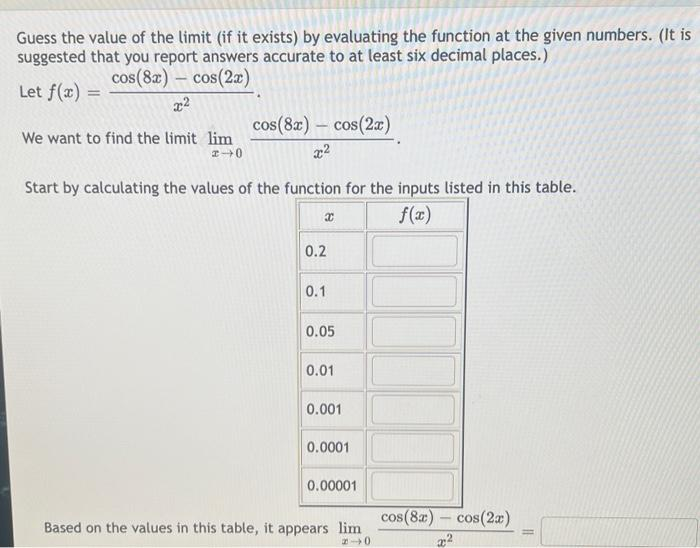Home / Expert Answers / Calculus / need-help-solving-nbsp-how-to-input-on-calculator-thanks-nbsp-guess-the-value-of-the-limit-if-it-pa896

# (Solved): need help solving how to input on calculator? thanks  Guess the value of the limit (if it ...

need help solving
how to input on calculator? thanksGuess the value of the limit (if it exists) by evaluating the function at the given numbers. (It is suggested that you report answers accurate to at least six decimal places.) Let $$f(x)=\frac{\cos (8 x)-\cos (2 x)}{x^{2}}$$ We want to find the limit $$\lim _{x \rightarrow 0} \frac{\cos (8 x)-\cos (2 x)}{x^{2}}$$. Start by calculating the values of the function for the inputs listed in this table. Based on the values in this table, it appears $$\lim _{x \rightarrow 0} \frac{\cos (0 x)-\cos (2 x)}{x^{2}}=$$

We have an Answer from Expert